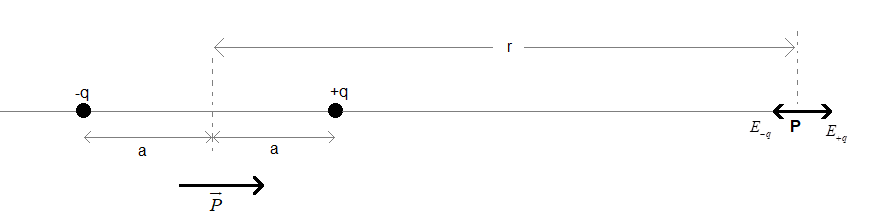Courses
Courses for Kids
Free study material
Free LIVE classes
MoreLIVE
Join Vedantu’s FREE Mastercalss

# Drive an expression for the electric field due to an electric dipole at a point on the axial line.Verified
336.6k+ views
Hint: Electric dipole is a pair of charges with equal magnitudes (q) that are ‘2a’ distance apart. Every dipole has a dipole moment equal to 2qa. Dipole moment is a vector denoted as $\overrightarrow{P}$.
Formula used:
$\overrightarrow{A}=A.\widehat{A}$
$E=\dfrac{kq}{{{r}^{2}}}$

Consider an electric dipole with a dipole moment $\overrightarrow{P}$as shown below. Magnitude of the dipole moment be $P=2qa$.The two charges on the dipole are 2a distance apart, as shown.

Let P be a point on the axial line of the dipole. Let the distance of point P from the midpoint of the dipole be r such that r>>>a.

The electric field at point P will be due to the two charges on the given dipole.

The magnitude of electric field due to the charge +q will be ${{E}_{+q}}=\dfrac{kq}{{{\left( r-a \right)}^{2}}}$.

Since it is a positive charge, the direction of electric field ${{E}_{+q}}$ will be along the direction of dipole moment (away from the charge).

The magnitude of electric field due to the charge -q will be ${{E}_{-q}}=\dfrac{kq}{{{\left( r+a \right)}^{2}}}$.

Since it is a negative charge, the direction of electric field ${{E}_{-q}}$ will be in the opposite direction of the dipole moment (towards the charge).

Therefore the net electric field at point P will be $E={{E}_{+q}}-{{E}_{-q}}$.

$\Rightarrow E=\dfrac{kq}{{{\left( r-a \right)}^{2}}}-\dfrac{kq}{{{\left( r+a \right)}^{2}}}$
$\Rightarrow E=kq\left( \dfrac{1}{{{\left( r-a \right)}^{2}}}-\dfrac{1}{{{\left( r+a \right)}^{2}}} \right)$
$\Rightarrow E=kq\left( \dfrac{{{\left( r+a \right)}^{2}}-{{\left( r-a \right)}^{2}}}{{{\left( r-a \right)}^{2}}.{{\left( r+a \right)}^{2}}} \right)$
$\Rightarrow E=kq\left( \dfrac{{{r}^{2}}+2ar+{{a}^{2}}-({{r}^{2}}-2ar+{{a}^{2}})}{{{\left( \left( r-a \right).\left( r+a \right) \right)}^{2}}} \right)$
$\Rightarrow E=kq\left( \dfrac{4ar}{{{\left( {{r}^{2}}-{{a}^{2}} \right)}^{2}}} \right)$
$\Rightarrow E=4kqa\left( \dfrac{r}{\left( {{r}^{4}}-2ar+{{a}^{4}} \right)} \right)$.
We can the above equation as,
$\Rightarrow E=2k(2qa)\left( \dfrac{r}{\left( {{r}^{4}}-2ar+{{a}^{4}} \right)} \right)$
And $2qa=P$

Therefore, $E=2kP\left( \dfrac{r}{\left( {{r}^{4}}-2ar+{{a}^{4}} \right)} \right)$ ………(i)
But, it is given that r is very much greater than a (r>>>a).

Therefore, in the expression $\left( {{r}^{4}}-2ar+{{a}^{4}} \right)$ of equation (i), the term $-2ar+{{a}^{4}}$ is very much smaller than the term ${{r}^{4}}$. When a very smaller number is added to a very large number, the sum will be almost equal to the larger number. Therefore, the smaller number does not contribute much to the sum and can be considered negligible.

Therefore, $\left( {{r}^{4}}-2ar+{{a}^{4}} \right)\approx {{r}^{4}}$.
Now we can write the equation (i) as, $E=2kP\left( \dfrac{r}{{{r}^{4}}} \right)$.
The final value of net electric field is $E=2kP\left( \dfrac{1}{{{r}^{3}}} \right)$.

In the vector form the net electric field will be written as $\overrightarrow{E}=2kP\left( \dfrac{1}{{{r}^{3}}} \right).\widehat{E}$, where $\widehat{E}$ is the unit vector of $\overrightarrow{E}$.

The direction of the field is in the direction of the dipole moment. Therefore, the unit vector of the net electric field and the dipole moment vectors will be equal i.e. $\widehat{E}=\widehat{P}$.

Now, the net electric is $\overrightarrow{E}=2kP\left( \dfrac{1}{{{r}^{3}}} \right).\widehat{P}$.

The product of the magnitude of a vector and its unit vector gives us the vector. Therefore, $P.\widehat{P}=\overrightarrow{P}$

This implies that $\overrightarrow{E}=2k\left( \dfrac{1}{{{r}^{3}}} \right).\overrightarrow{P}$
Hence, the electric field due to a dipole, at a given point on its axial line is $\overrightarrow{E}=\dfrac{2k}{{{r}^{3}}}\overrightarrow{P}$.

Note: You may be thinking about the condition on r that it should be very much greater than a. there is a valid reason for this condition. We study the concept of dipole because molecules exist in the form of dipoles. For example, the bond between oxygen atom and hydrogen atom in water molecules is a dipole moment. You may know that the distance between these atoms is very small and therefore it is negligible when compared to the distance r on its axial line, from its midpoint.

Last updated date: 23rd Sep 2023
Total views: 336.6k
Views today: 9.36k